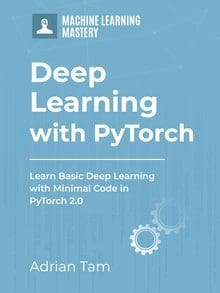# Using Dataset Classes in PyTorch

In machine learning and deep learning problems, a lot of effort goes into preparing the data. Data is usually messy and needs to be preprocessed before it can be used for training a model. If the data is not prepared correctly, the model won’t be able to generalize well.
Some of the common steps required for data preprocessing include:

• Data normalization: This includes normalizing the data between a range of values in a dataset.
• Data augmentation: This includes generating new samples from existing ones by adding noise or shifts in features to make them more diverse.

Data preparation is a crucial step in any machine learning pipeline. PyTorch brings along a lot of modules such as torchvision which provides datasets and dataset classes to make data preparation easy.

In this tutorial we’ll demonstrate how to work with datasets and transforms in PyTorch so that you may create your own custom dataset classes and manipulate the datasets the way you want. In particular, you’ll learn:

• How to create a simple dataset class and apply transforms to it.
• How to build callable transforms and apply them to the dataset object.
• How to compose various transforms on a dataset object.

Note that here you’ll play with simple datasets for general understanding of the concepts while in the next part of this tutorial you’ll get a chance to work with dataset objects for images.

Kick-start your project with my book Deep Learning with PyTorch. It provides self-study tutorials with working code.

Let’s get started.Using Dataset Classes in PyTorch
Picture by NASA. Some rights reserved.

## Overview

This tutorial is in three parts; they are:

• Creating a Simple Dataset Class
• Creating Callable Transforms
• Composing Multiple Transforms for Datasets

## Creating a Simple Dataset Class

Before we begin, we’ll have to import a few packages before creating the dataset class.

We’ll import the abstract class Dataset from torch.utils.data. Hence, we override the below methods in the dataset class:

• __len__ so that len(dataset) can tell us the size of the dataset.
• __getitem__ to access the data samples in the dataset by supporting indexing operation. For example, dataset[i] can be used to retrieve i-th data sample.

Likewise, the torch.manual_seed() forces the random function to produce the same number every time it is recompiled.

Now, let’s define the dataset class.

In the object constructor, we have created the values of features and targets, namely x and y, assigning their values to the tensors self.x and self.y. Each tensor carries 20 data samples while the attribute data_length stores the number of data samples. Let’s discuss about the transforms later in the tutorial.

The behavior of the SimpleDataset object is like any Python iterable, such as a list or a tuple. Now, let’s create the SimpleDataset object and look at its total length and the value at index 1.

This prints

As our dataset is iterable, let’s print out the first four elements using a loop:

This prints

## Creating Callable Transforms

In several cases, you’ll need to create callable transforms in order to normalize or standardize the data. These transforms can then be applied to the tensors. Let’s create a callable transform and apply it to our “simple dataset” object we created earlier in this tutorial.

We have created a simple custom transform MultDivide that multiplies x with 2 and divides y by 3. This is not for any practical use but to demonstrate how a callable class can work as a transform for our dataset class. Remember, we had declared a parameter transform = None in the simple_dataset. Now, we can replace that None with the custom transform object that we’ve just created.

So, let’s demonstrate how it’s done and call this transform object on our dataset to see how it transforms the first four elements of our dataset.

This prints

As you can see the transform has been successfully applied to the first four elements of the dataset.

### Want to Get Started With Deep Learning with PyTorch?

Take my free email crash course now (with sample code).

Click to sign-up and also get a free PDF Ebook version of the course.

## Composing Multiple Transforms for Datasets

We often would like to perform multiple transforms in series on a dataset. This can be done by importing Compose class from transforms module in torchvision. For instance, let’s say we build another transform SubtractOne and apply it to our dataset in addition to the MultDivide transform that we have created earlier.

Once applied, the newly created transform will subtract 1 from each element of the dataset.

As specified earlier, now we’ll combine both the transforms with Compose method.

Note that first MultDivide transform will be applied onto the dataset and then SubtractOne transform will be applied on the transformed elements of the dataset.
We’ll pass the Compose object (that holds the combination of both the transforms i.e. MultDivide() and SubtractOne()) to our SimpleDataset object.

Now that the combination of multiple transforms has been applied to the dataset, let’s print out the first four elements of our transformed dataset.

Putting everything together, the complete code is as follows:

## Summary

In this tutorial, you learned how to create custom datasets and transforms in PyTorch. Particularly, you learned:

• How to create a simple dataset class and apply transforms to it.
• How to build callable transforms and apply them to the dataset object.
• How to compose various transforms on a dataset object.

## Get Started on Deep Learning with PyTorch!#### Learn how to build deep learning models

...using the newly released PyTorch 2.0 library

Discover how in my new Ebook:
Deep Learning with PyTorch

It provides self-study tutorials with hundreds of working code to turn you from a novice to expert. It equips you with
tensor operation, training, evaluation, hyperparameter optimization, and much more...